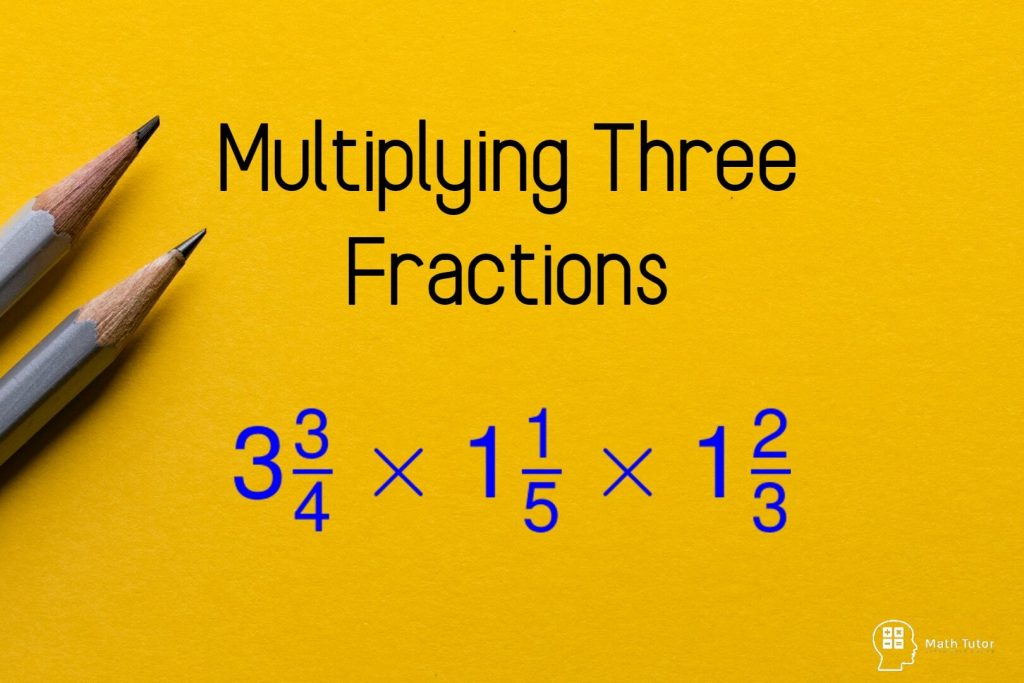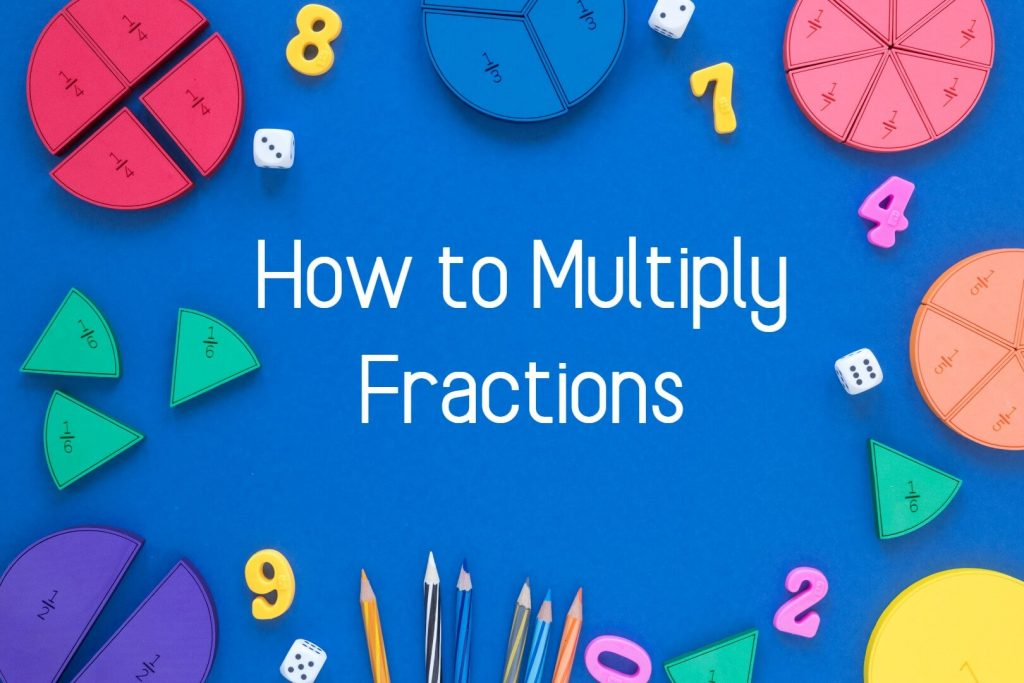# Fractions

All fractions related math lessons, examples, exercises, Worksheets available. Learn math easy and fun way.## Multiplying Three Fractions

In this lesson, we are going to learn how to multiplying three fractions.We have discussed how to multiply fractions from basics in another lesson. You can visit the following link to view the previous lesson.How to Multiply Fractions Multiplying Three Fractions Multiplying three fractions seems a little more complicated than multiplying two fractions. But the …